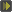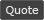WoWInterface - View Single Post - oUF PP
 Thread: oUF PP View Single Post
08-20-16, 07:09 AM   #20
 Originally Posted by FreebaserAdding a check for r and returning a value should fix that. Update the hex function to this. Code: ```local function hex(r, g, b) if(not r) then return "|cffFFFFFF" end if(type(r) == 'table') then if(r.r) then r, g, b = r.r, r.g, r.b else r, g, b = unpack(r) end end if(r > 1) then return ('|cff%02x%02x%02x'):format(r, g, b) else return ('|cff%02x%02x%02x'):format(r * 255, g * 255, b * 255) end end```